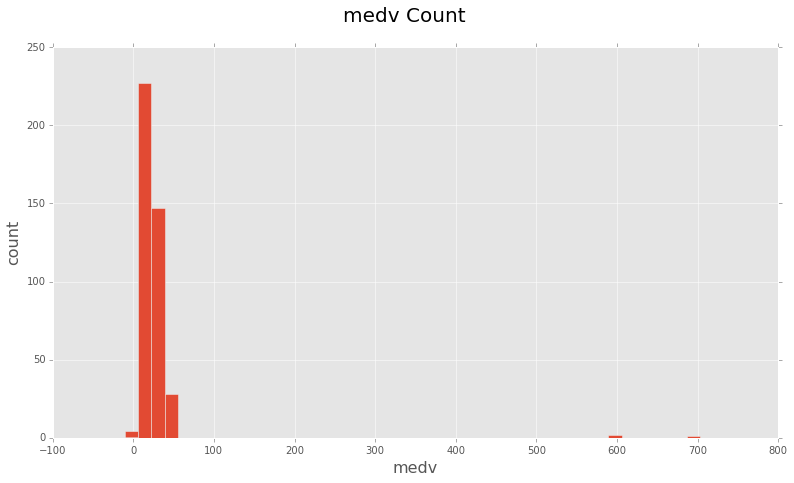Least absolute deviations(L1) and Least square errors(L2) are the two standard loss functions, that decides what function should be minimized while learning from a dataset.

L1 Loss function minimizes the absolute differences between the estimated values and the existing target values. So, summing up each target </span> value $$y_i$$ and corresponding estimated value $$h(x_i)$$, where $$x_i$$ denotes the feature set of a single sample, Sum of absolute differences for ‘n’ samples can be calculated as,

\begin{align*} & S = \sum_{i=0}^n|y_i - h(x_i)| \end{align*}

On the other hand, L2 loss function minimizes the squared differences between the estimated and existing target values.

\begin{align*} & S = \sum_{i=0}^n(y_i - h(x_i))^2 \end{align*}

As apparent from above formulae that L2 error will be much larger in the case of outliers compared to L1. Since, the difference between an incorrectly predicted target value and original target value will be quite large and squaring it will make it even larger.

As a result, L1 loss function is more robust and is generally not affected by outliers. On the contrary L2 loss function will try to adjust the model according to these outlier values, even on the expense of other samples. Hence, L2 loss function is highly sensitive to outliers in the dataset.

We’ll see how outliers can affect the performance of a regression model. We are going to use pandas, scikit-learn and numpy to work through this. I’d highly recommend to have a look at the ipython notebook containing the code on this post.

We’ll be using Boston Housing Prices dataset and will to try to predict the prices using Gradient Boosting Regressor from scikit-learn. You can downloaded the dataset directly from UCI Datasets or from this csv.

We are goint to start with reading the data from the csv file.

#### Regression without any Outliers:

At this moment, our housing dataset is pretty much clean and doesn’t contain any outliers as such. So let’s fit a GB regressor with L1 and L2 loss functions.

With a L1 loss function and no outlier we get a value of RMSE: 3.440147. Let’s see what results we get with L2 loss function.

This prints out a mean squared value of RMSE -> 2.542019.

As apparent from RMSE errors of L1 and L2 loss functions, Least Squares(L2) outperform L1, when there are no outliers in the data.

#### Regression with Outliers:

After looking at the minimum and maximum values of ‘medv’ column, we can see that the range of values in ‘medv’ is [5, 50].
Let’s add a few Outliers in this Dataset, so that we can see some significant differences with L1 and L2 loss functions.

Now, we are going to generate 5 random samples, such that their values lies in the [min, max] range of respective features.

array([[ 17.04578252, 19.15194504, 5.68465061, 0.19151945, 0.47807845, 4.56054001, 21.49653863, 3.23572024, 5.40494736, 287.356192 , 14.40028283, 76.27278363, 8.67066488],…, [ 69.40067405, 77.99758081, 21.73774005, 0.77997581, 0.76406824, 7.63169374, 78.63565097, 9.70691596, 18.93944359, 595.70732345, 19.9317726 , 309.64280598, 29.99632329]])You can see there are some clear outliers at 600, 700 and even one or two ‘medv’ values are 0.
Since, our outliers are in place now, we will once again fit the GradientBoostingRegressor with L1 and L2 Loss functions to see the contrast in their performances with outliers.

We get a RMSE value of 7.055568, with L1 loss function and existing outliers.

On the other hand, we get a RMSE value of 9.806251, with L2 loss function and existing outliers.

With outliers in the dataset, a L2(Loss function) tries to adjust the model according to these outliers on the expense of other good-samples, since the squared-error is going to be huge for these outliers(for error > 1). On the other hand L1(Least absolute deviation) is quite resistant to outliers.
As a result, L2 loss function may result in huge deviations in some of the samples which results in reduced accuracy.

So, if you can ignore the ouliers in your dataset or you need them to be there, then you should be using a L1 loss function, on the other hand if you don’t want undesired outliers in the dataset and would like to use a stable solution then first of all you should try to remove the outliers and then use a L2 loss function. Or performance of a model with a L2 loss function may deteriorate badly due to the presence of outliers in the dataset.

Whenever in doubt, prefer L2 loss function, it works pretty well in most of the situations.